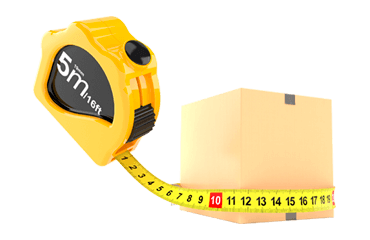EN# Volume Calculator

When calculating the cost of delivery, it is necessary to note that for tariffing two indicators of the weight of the parcel are taken into account - actual and volumetric. Actual weight is calculated by weighing the parcel on the scale. Volumetric weight is determined based on the sizes of the parcel and the value of the volumetric weight coefficient of the selected Delivery Service.
If your parcel is lightweight, but voluminous, then before sending you can independently calculate the value of the volumetric weight in just three steps:
1. Measure the height, length and width of the parcel in centimeters
2. Multiply the resulting values ​​by the formula: Length x Height x Width
3. Divide the resulting value by the volumetric weight coefficient of the selected Delivery Service
The resulting value is the volumetric weight of your parcel. For example:
• The parcel must be sent by the Delivery Service with the volumetric weight coefficient of 6000.
• The parcel length is 45 cm, height is 35 cm, width is 40 cm.
• Volumetric weight will be calculated by the formula:
$\frac{45х35х40}{6000}=10,5$
• The volumetric weight of the parcel is 10.5 kg.To check which weight indicator will be used as the basis for the calculation, it is necessary to compare both the obtained weight values. A higher value will be the main one when calculating the tariff for sending the parcel. For example:
Item
Actual weight
Volumetric weight
 Parcel A* 1.34 2.46 Parcel B** 14.3 13.1
* When sending Parcel A, the shipping cost will be calculated based on the value of the volumetric weight, since it exceeds the value of the actual weight.
** When sending Parcel B, the shipping cost will be calculated based on the value of the actual weight, since it exceeds the value of the volumetric weight.